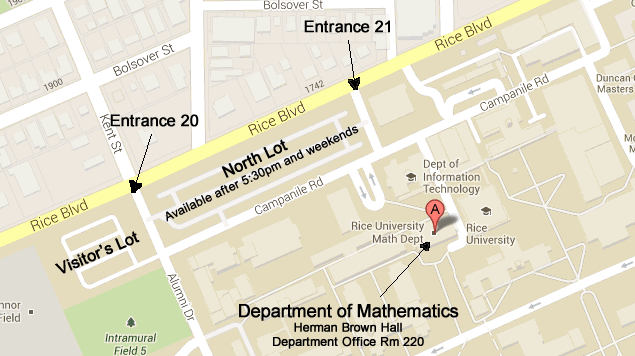# Which Courses Should I Take?

#### Science-Engineering Calculus Sequence:

• MATH 101 - Single Variable Calculus I (3 hours)
• MATH 102 - Single Variable Calculus II (3 hours)
• MATH 211 - Ordinary Differential Equations (3 hours)
• MATH 212 - Multivariable Calculus (3 hours)

These four courses should be taken in sequence, although 211 and 212 are interchangeable. Each of these courses is offered every semester.

The Math Department recommends that students enroll in as advanced a course as they might be prepared for, rather than repeating material, even if you do not have university credit for something. Many majors will allow students to substitute higher math courses in place of a course like Math 101 or 102. Check with your major department for more information.

If you have learned the material in Math 101 prior to enrolling at Rice, but do not have credit for it via an AP Calculus Exam (or similar), you may be able to earn course credit by taking a Rice exam. See this page for more details.

#### Honors Calculus Courses:

• MATH 220 - Honors Differential Equations (Spring) (3 hours)
• MATH 232 - Honors Multivariable Calculus (Spring) (3 hours)

Students should consider the honors calculus courses if they have a strong math background and are either considering a major in an area with a substantial math component, or just enjoy a challenge and want to go beyond just learning to solve problems. All of these courses will teach students how to prove mathematical statements.

Math 232 covers all of the material in Math 212, but also stresses theoretical aspects of multivariable calculus. It goes beyond Math 212 in that it covers calculus in arbitrary dimensions, not just 2- and 3-dimensions. Math 232 also has a linear algebra prerequisite. Math 354 is the ideal course to take before enrolling in Math 232, but Math 355 can also work, especially if enrolling in Math 290 (Mathematical Writing Seminar) concurrently.

Math 220 is offered in the spring and satisfies requirements for Math 211. It covers the same material, but with more of an emphasis on theory.

Students enrolling in their first proofs course should also consider concurrently enrolling in Math 290 (Mathematical Writing Seminar), which is a 1-credit, half-semester course that aims to provide additional targeted feedback on students' mathematical writing.

#### Other Calculus Courses (for Distribution):

• MATH 111 - Calculus: Differentiation and its Applications (Fall) (3 hours)
• MATH 112 - Calculus: Integration and its Applications (Spring) (3 hours)

These courses emphasize problem solving, and do not go as far as 101-102. They are not intended for science or engineering majors, but a student may take 111, 112, and then 102 (or 111, 101, and 102).

#### Dropback Option:

Students may drop back from a more advanced math class to a less advanced one at any point in the first seven weeks of the semester, per the chart below. Contact your instructor or a math advisor for more information.

From To
Honors Multivariable (Math 232) Multivariable Calc. (Math 212)
Honors Diff. Eqns. (Math 220) Diff. Eqns. (Math 211)
Honors Linear Algebra (Math 354) Linear Algebra (Math 355)
Multivariable Calc. (212) / Diff. Eqns. (211) Calculus II (Math 102)
Calculus II (Math 102) Calculus I (Math 101)
Calculus I (Math 101) Calculus: Differentiation (Math 111)

Students who have already taken some or all of the above courses should visit this page . Speaking to a MATH advisor may be the best way to decide which course to take.Large Map

Tel (713) 348-4829